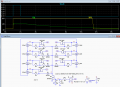# Using a capacitor to momentarly charge and switch on transistors

#### crutschow

Joined Mar 14, 2008
23,756
No that circuit won't work.
The base resistors must be grounded to turn on the PNP's, not connected to the battery.
And with 1kΩ resistors in parallel, the equivalent resistance is 1k/7 ≈ 143Ω, so to get a time-constant in the neighborhood of 10 seconds would require a capacitor of 10/143 = 70,000μFWhy did you think 5μF would do it?

And if the Fx's are the load for the batteries and the batteries are positive polarity, then you have the transistor emitters and collectors reversed.

What's the purpose of the diode?

What is the battery voltage?

How much current do the transistors need to carry?

Using P-MOSFET's might work better than the PNP's.

Last edited:

#### anishkgt

Joined Mar 21, 2017
387
The voltage is from 4.2v Li-ion. F1 - F7 are after a fuse and the load here are the cells named BAT_1 to BAT_7. The current would be less than 100ma (not tested yet) but based on the LM3194 and 70 Leds running 1mA it would sum up to 72mA.

The BAT_2 and others would be between the positive and negative terminals of the battery. Basically the idea is siwtch on the LM3914s momentarily for a couple of seconds till the cap discharged.

#### Attachments

• 160.7 KB Views: 2

#### crutschow

Joined Mar 14, 2008
23,756
Here's the LTspice simulation of a switching circuit for the seven batteries that should do what you want.
I used P-MOSFETs instead of the PNPs to avoid the small ON offset voltage that BJT's have.
The diodes are to isolate the gates when off (not needed for the highest voltage at M2).
Note the resistors R3, R4, and R7 to limit the ON Vgs voltage for the higher voltage test points.
The P-MOSFETs can be just about any that have at least a 50V rating and are logic-level types (Vgs max threshold ≤2V).

The outputs stay on (F1 output shown, yellow trace) for about 10 seconds after the switch is released, for the values of C1 and R8 shown.•anishkgt

#### anishkgt

Joined Mar 21, 2017
387

#### crutschow

Joined Mar 14, 2008
23,756
Would this P-CH MOSFET 60V 300MA SOT-23 work to switch the cells ? and M3 MOSFET N-CH 60V 0.2A
Those should work, but the 2.5Ω * 72mA = 180mV drop in the measured battery voltage due to the P-CH's ON-resistance seems a little problematic.
Suggest you find one with a lower on-resistance, probably one with less than an ohm.
Why does M4, M6, M7, M8 not require a voltage divider ?
The voltage divider is to keep the ON Vgs voltage below the typical MOSFET maximum of 20V.
If the supply voltage to ground is below 20V, then it doesn't need the divider.

I did notice an error in your schematic.
IC7 has the input accidentally connected to ground instead of the battery voltage.

•anishkgt

#### anishkgt

Joined Mar 21, 2017
387
Those should work, but the 2.5Ω * 72mA = 180mV drop in the measured battery voltage due to the P-CH's ON-resistance seems a little problematic.
Suggest you find one with a lower on-resistance, probably one with less than an ohm.
These cells can be supply max 3A but would not pull that much at anytime so i guess this would be better in terms of lowest voltage drop MOSFET P-CH 30V 3.8A SOT23 it has 65mΩ at 3.8A, 10v.

The voltage divider is to keep the ON Vgs voltage below the typical MOSFET maximum of 20V.
If the supply voltage to ground is below 20V, then it doesn't need the divider.
Thank you.

I did notice an error in your schematic.
IC7 has the input accidentally connected to ground instead of the battery voltage.
Missed that out completely, Thank you for pointing it out.

#### crutschow

Joined Mar 14, 2008
23,756
i guess this would be better in terms of lowest voltage drop MOSFET P-CH 30V 3.8A SOT23 it has 65mΩ at 3.8A, 10v
Much better.
The would give only a 72mA * 65mΩ =4.7mV error in the measured voltage.

•anishkgt